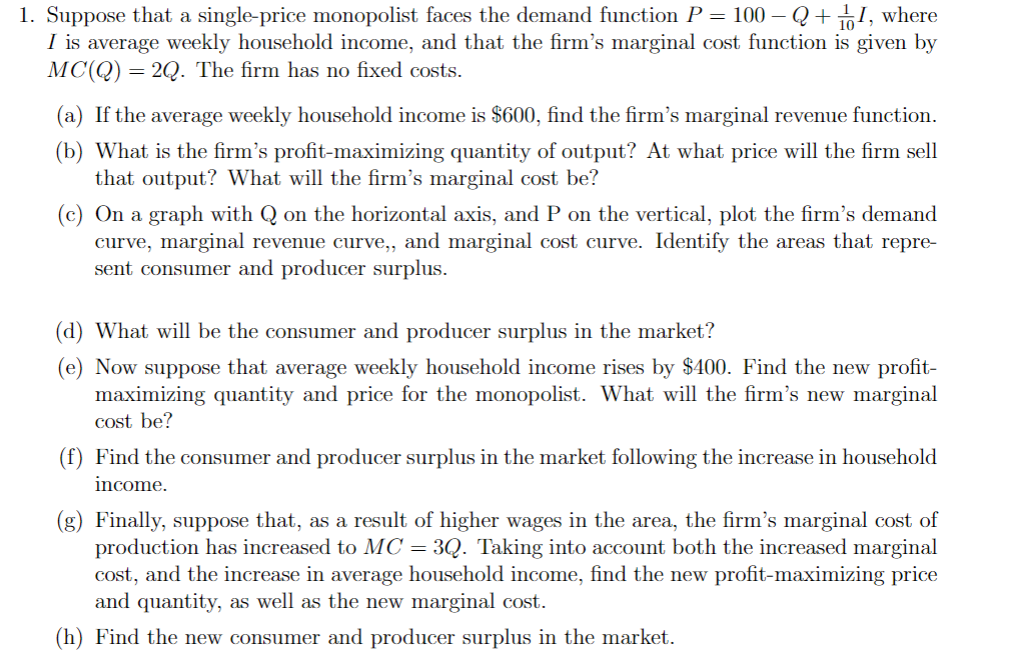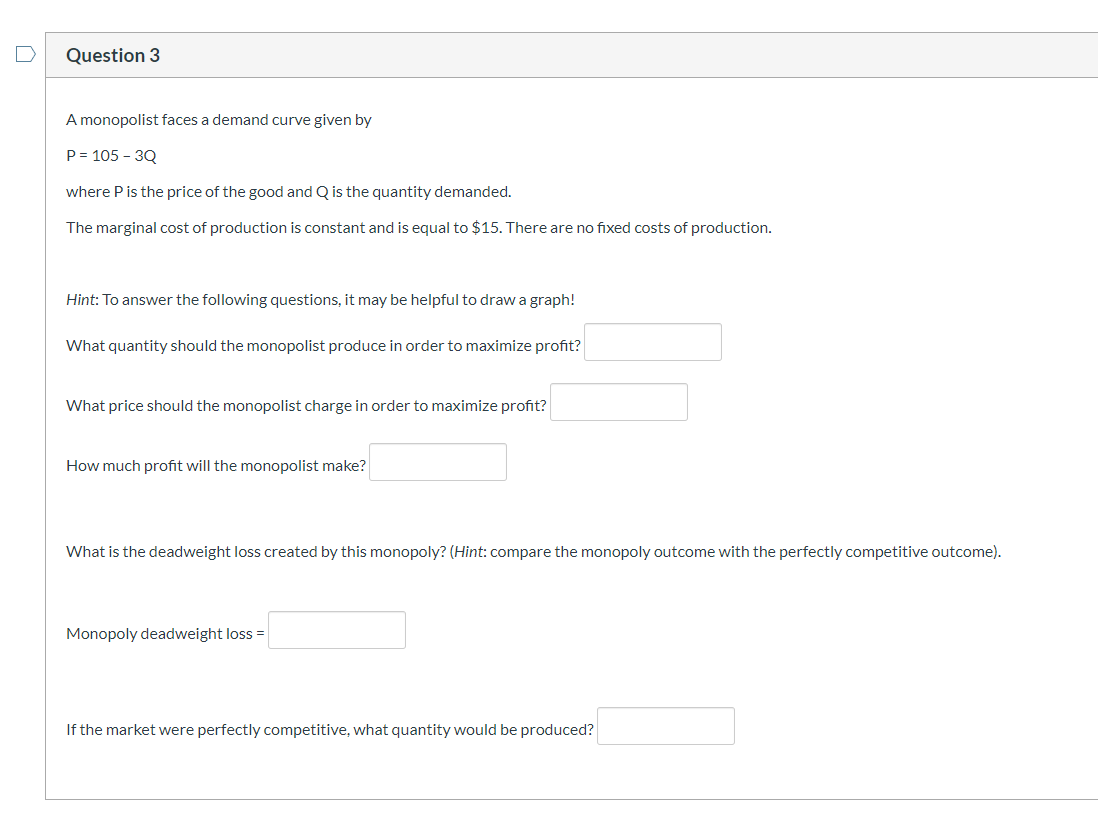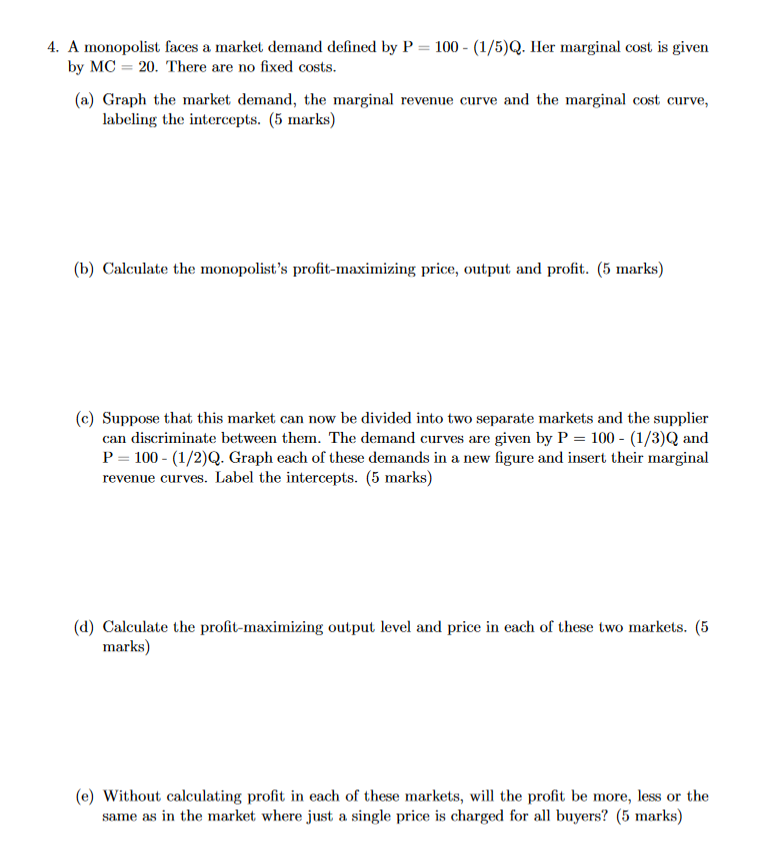Question

A monopolist has variable costs of VC = q2 and faces a demand curve of P = 24 – q, where P is price and q the quantity sold. If the monopolist sets a single price what is profit (assume there are no fixed costs)?

A monopolist has variable costs of VC = q2 and faces a demand curve of P = 24 – q, where P is price and q the quantity sold. If the monopolist sets a single price what is profit (assume there are no fixed costs)?

We need at least 10 more requests to produce the answer.

0 / 10 have requested this problem solution

The more requests, the faster the answer.

All students who have requested the answer will be notified once they are available.

Earn Coins

Coins can be redeemed for fabulous gifts.

Similar Homework Help Questions
• Q2) A monopolist has variable costs of VC = q2 and faces a demand curve of P = 24 – q, where P is price and q the quantity sold. If the monopolist sets a single price, what is the resulting loss in th...

Q2) A monopolist has variable costs of VC = q2 and faces a demand curve of P = 24 – q, where P is price and q the quantity sold. If the monopolist sets a single price, what is the resulting loss in the gains from trade? a) \$8 b) \$6 c) \$12 d) \$4 e) None of the above

• Suppose a monopolist faces the constant price elasticity demand curve: p = Q? where ? <...

Suppose a monopolist faces the constant price elasticity demand curve: p = Q? where ? < 0. The monopolist has a constant marginal cost of c. a. If ? < -1, can you determine what price and quantity will the monopolist set? Explain. b. If 0>?>-1, what is the price and quantity the monopolist will set?

• 1. Suppose that a single-price monopolist faces the demand function P 100 Q where I is...1. Suppose that a single-price monopolist faces the demand function P 100 Q where I is average weekly household income, and that the firm's marginal cost function is given by MC(Q) 2Q. The firm has no fixed costs. = (a) If the average weekly household income is \$600, find the firm's marginal revenue function. (b) What is the firm's profit-maximizing quantity of output? At what price will the firm sell that output? What will the firm's marginal cost be? (c)...

• Question 3 A monopolist faces a demand curve given by P = 105 - 30 where...Question 3 A monopolist faces a demand curve given by P = 105 - 30 where P is the price of the good and Q is the quantity demanded. The marginal cost of production is constant and is equal to \$15. There are no fixed costs of production. Hint: To answer the following questions, it may be helpful to draw a graph! What quantity should the monopolist produce in order to maximize profit? What price should the monopolist charge in...

• A monopolist faces a demand curve given by P=70-2Qwhere P is the price of the good...

A monopolist faces a demand curve given by P=70-2Qwhere P is the price of the good and Q is the quantity demanded, the marginal cost of production is constant and is equal to \$6, there are no fixed cost, how much profit will the monopolist make?

• (18 points) A monopolist faces a demand curve given by P = 200 – 10Q, where...

(18 points) A monopolist faces a demand curve given by P = 200 – 10Q, where P is the price of the good and Q is the quantity demanded.  The marginal cost of production is constant and is equal to \$60.  There are no fixed costs of production. A) (4 points) What quantity should the monopolist produce in order to maximize profit? B) (2 points) What price should the monopolist charge in order to maximize profit? C) (4 points) How much profit...

• 3.  (18 points) A monopolist faces a demand curve given by: P = 200 – 10Q, where...

3.  (18 points) A monopolist faces a demand curve given by: P = 200 – 10Q, where P is the price of the good and Q is the quantity demanded.  The marginal cost of production is constant and is equal to \$60.  There are no fixed costs of production. A) (4 points) What quantity should the monopolist produce in order to maximize profit? B) (2 points) What price should the monopolist charge in order to maximize profit? C) (4 points) How much profit...

• A monopolist faces a market demand curve given by

A monopolist faces a market demand curve given by Q=70-P a. If the monopolist can produce at constant average and marginal costs ofAC-MC-6, what output level will the monopolist choose to maximize profits? What is the price at this output level? What are the monopolist's profits? b. Assume instead that the monopolist has a cost structure where total costs are described by C(Q) = 0.25Q2 - 5Q + 300. With the monopolist facing the same market demand and marginal revenue, what price-quantity combination will be chosen now...

• 4. A monopolist faces a market demand defined by P 20. There are no fixed costs. 100 (1/5)Q. Her ...4. A monopolist faces a market demand defined by P 20. There are no fixed costs. 100 (1/5)Q. Her marginal cost is given by MC (a) Graph the market demand, the marginal revenue curve and the marginal cost curve, labeling the intercepts. (5 marks) (b) Calculate the monopolist's profit-maximizing price, output and profit. (5 marks) (c) Suppose that this market can now be divided into two separate markets and the supplier can discriminate between them. The demand curves are given...

• A monopolist has a cost curve c(q) = q^2-12q+8 and faces an inverse demand curve p(q) = 80-20q. Find the monopolist pric...

A monopolist has a cost curve c(q) = q^2-12q+8 and faces an inverse demand curve p(q) = 80-20q. Find the monopolist price and quantity, (p,q).